# Technical Indicators in Xamarin Charts (SfChart)

24 Nov 202224 minutes to read

The different types of technical indicators available in chart are follows:

## Adding technical indicators to chart

The following section illustrates how to add technical indicators to chart.

Initializing indicator

Create an instance for any technical indicator, and add it to the `TechnicalIndicators` collection.

Here, for an instance, the `AccumulationDistributionIndicator` is added.

``````<chart:SfChart>
...
<chart:SfChart.TechnicalIndicators>
<chart:AccumulationDistributionIndicator />
</chart:SfChart.TechnicalIndicators>

</chart:SfChart>``````
``````SfChart chart = new SfChart();
...
AccumulationDistributionIndicator indicator= new AccumulationDistributionIndicator();

Binding data

Set the `ItemsSource` and binding paths (`Open`, `High`, `Low`, `Close`, `XBindingPath` and `Trigger`) to fetch the values from model.

``````<chart:SfChart>
...
<chart:SfChart.TechnicalIndicators>
<chart:AccumulationDistributionIndicator ItemsSource="{Binding TechnicalIndicatorData}" XBindingPath="XValue" Open="Open" High="High" Low="Low" Close="Close" Volume="Volume"/>
</chart:SfChart.TechnicalIndicators>

</chart:SfChart>``````
``````SfChart chart = new SfChart()
{
...
TechnicalIndicators =
{
new AccumulationDistributionIndicator()
{
ItemsSource = viewModel.TechnicalIndicatorData,
XBindingPath = "XValue",
Open = "Open",
High = "High",
Low = "Low",
Close = "Close"
}
}
};``````

Binding the items source of chart series

By setting `Name` property of `FinancialSeriesBase` to the `SeriesName` property of `FinancialTechnicalIndicator` you can bind the items source of chart series to technical indicators, including x and y axis.

``````<chart:SfChart>
...
<chart:SfChart.Series>
<chart:HiLoOpenCloseSeries Name="OHLC" ItemsSource="{Binding TechnicalIndicatorData}" XBindingPath="XValue" Open="Open" High="High" Low="Low" Close="Close"/>
</chart:SfChart.Series>
<chart:SfChart.TechnicalIndicators>
<chart:AccumulationDistributionIndicator SeriesName="OHLC"/>
</chart:SfChart.TechnicalIndicators>

</chart:SfChart>``````
``````SfChart chart = new SfChart()
{
...
Series =
{
new HiLoOpenCloseSeries()
{
ItemsSource = viewModel.TechnicalIndicatorData,
XBindingPath = "XValue",
Open = "Open",
High = "High",
Low = "Low",
Close = "Close",
Name = "OHLC"
}
},

TechnicalIndicators =
{
new AccumulationDistributionIndicator()
{
SeriesName = "OHLC"
}
}
};``````

Technical indicators have the below properties as common;

The `XAxis` and `YAxis` properties of technical indicators are used to set the x and y-axes.

You can define the axis using the following code example.

``````<chart:SfChart>
...
<chart:SfChart.TechnicalIndicators>
<chart:AccumulationDistributionIndicator SeriesName="OHLC">
<chart:AccumulationDistributionIndicator.XAxis>
<chart:NumericalAxis/>
</chart:AccumulationDistributionIndicator.XAxis>
</chart:AccumulationDistributionIndicator>
</chart:SfChart.TechnicalIndicators>

</chart:SfChart>``````
``````SfChart chart = new SfChart()
{
...
TechnicalIndicators =
{
new AccumulationDistributionIndicator()
{
SeriesName = "OHLC",
XAxis = new NumericalAxis()
}
}
};``````

Animation

`SfChart` provides animation support for technical indicators. Technical indicators will be animated whenever the ItemsSource changes. Animation can be enabled by setting the `EnableAnimation` property to true. You can also control the duration of the animation using the `AnimationDuration` property.

``````<chart:SfChart>
...
<chart:SfChart.TechnicalIndicators>
<chart:AccumulationDistributionIndicator SeriesName="OHLC" EnableAnimation="True" AnimationDuration="0.8"/>
</chart:SfChart.TechnicalIndicators>

</chart:SfChart>``````
``````SfChart chart = new SfChart()
{
...
TechnicalIndicators =
{
new AccumulationDistributionIndicator()
{
SeriesName = "OHLC",
EnableAnimation = true,
AnimationDuration = 0.8
}
}
};``````

## Indicator visibility

The `IsVisible` property of `FinancialTechnicalIndicator` is used to controls the visibility of the indicator.

``````<chart:SfChart>
...
<chart:SfChart.TechnicalIndicators>
<chart:AccumulationDistributionIndicator SeriesName="OHLC" IsVisible="False"/>
</chart:SfChart.TechnicalIndicators>

</chart:SfChart>``````
``````SfChart chart = new SfChart()
{
...
TechnicalIndicators =
{
new AccumulationDistributionIndicator()
{
SeriesName = "OHLC",
IsVisible = false
}
}
};``````

## Average true range indicator

ATR indicator is a technical analysis volatility indicator. This indicator does not provide an indication of price trend; simply the degree of price volatility. The average true range is an N-day smoothed moving average (SMMA) of the true range values.

You can define the `AverageTrueIndicator` using the following code example.

``````<chart:SfChart>
...
<chart:SfChart.TechnicalIndicators>
<chart:AverageTrueIndicator ItemsSource="{Binding TechnicalIndicatorData}" Period="14" SignalLineColor="Blue" XBindingPath="XValue" High="High" Low="Low" Open="Open" Close="Close"/>
</chart:SfChart.TechnicalIndicators>

</chart:SfChart>``````
``````SfChart chart = new SfChart()
{
...
TechnicalIndicators =
{
new AverageTrueIndicator()
{
Period = 14,
SignalLineColor = Color.Blue,
ItemsSource = viewModel.TechnicalIndicatorData,
XBindingPath = "XValue",
Open = "Open",
High = "High",
Low = "Low",
Close = "Close",
}
}
};``````

The following screenshot illustrates an ATR indicator.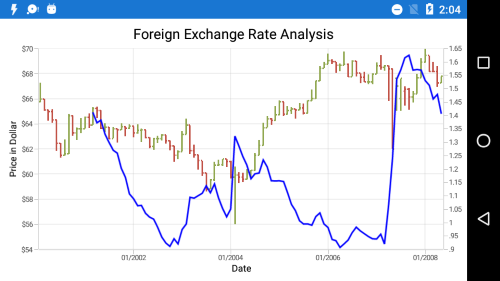## Simple moving average indicator

A SMA indicator is a simple, arithmetic moving average that is calculated by adding the closing price for number of time periods and dividing the total value by the number of time periods.

The following code example demonstrates the usage of `SimpleMovingAverageIndicator`.

``````<chart:SfChart>
...
<chart:SfChart.TechnicalIndicators>
<chart:SimpleMovingAverageIndicator ItemsSource="{Binding TechnicalIndicatorData}" Period="14" SignalLineColor="Blue" XBindingPath="XValue" High="High" Low="Low" Open="Open" Close="Close"/>
</chart:SfChart.TechnicalIndicators>

</chart:SfChart>``````
``````SfChart chart = new SfChart()
{
...
TechnicalIndicators =
{
new SimpleMovingAverageIndicator()
{
Period = 14,
SignalLineColor = Color.Blue,
ItemsSource = viewModel.TechnicalIndicatorData,
XBindingPath = "XValue",
Open = "Open",
High = "High",
Low = "Low",
Close = "Close",
}
}
};``````

The following screenshot illustrates an SMA indicator.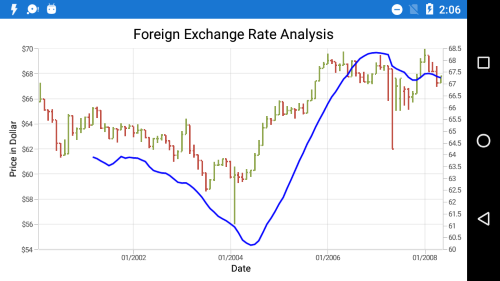## Relative strength index indicator

The RSI indicator has additional two lines other than signal line; they indicate the overbought and oversold region.

The `UpperLineColor` property is used to define the color for the line that indicates overbought region, and the `LowerLineColor` property is used to define the color for the line that indicates oversold region.

To define the `RSITechnicalIndicator`, use the following code example.

``````<chart:SfChart>
...
<chart:SfChart.TechnicalIndicators>
<chart:RSIIndicator ItemsSource="{Binding TechnicalIndicatorData}" Period="14" SignalLineColor="Blue" UpperLineColor="Teal" LowerLineColor="Red" XBindingPath="XValue" High="High" Low="Low" Open="Open" Close="Close"/>
</chart:SfChart.TechnicalIndicators>

</chart:SfChart>``````
``````SfChart chart = new SfChart()
{
...
TechnicalIndicators =
{
new RSIIndicator()
{
Period = 14,
SignalLineColor = Color.Blue,
UpperLineColor = Color.Teal,
LowerLineColor = Color.Red,
ItemsSource = viewModel.TechnicalIndicatorData,
XBindingPath = "XValue",
Open = "Open",
High = "High",
Low = "Low",
Close = "Close",
}
}
};``````

The following screenshot illustrates an RSI technical indicator.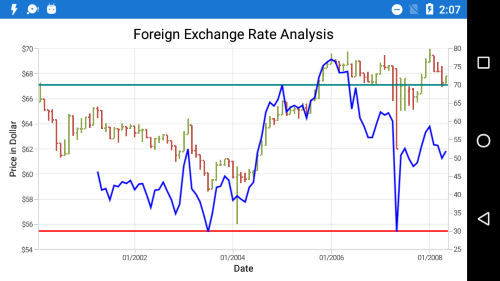## Accumulation distribution indicator

Accumulation distribution indicator is a volume-based indicator designed to measure the accumulative flow of money into and out of a security. It requires `Volume` property additionally with the data source to calculate the signal line.

The following code example helps you to add `AccumulationDistributionIndicator`.

``````<chart:SfChart>
...
<chart:SfChart.TechnicalIndicators>
<chart:AccumulationDistributionIndicator ItemsSource="{Binding TechnicalIndicatorData}" SignalLineColor="Blue" XBindingPath="XValue" Open="Open" High="High" Low="Low" Close="Close" Volume="Volume"/>
</chart:SfChart.TechnicalIndicators>

</chart:SfChart>``````
``````SfChart chart = new SfChart()
{
...
TechnicalIndicators =
{
new AccumulationDistributionIndicator()
{
SignalLineColor = Color.Blue,
ItemsSource = viewModel.TechnicalIndicatorData,
XBindingPath = "XValue",
Open = "Open",
High = "High",
Low = "Low",
Close = "Close",
Volume = "Volume"
}
}
};``````

The following screenshot illustrates an accumulation distribution indicator.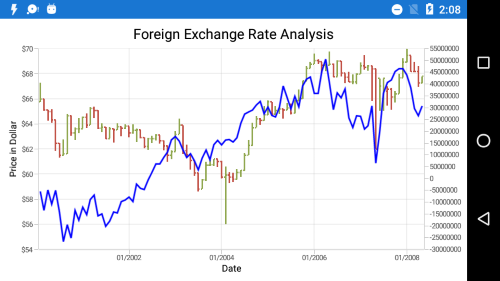## Momentum indicator

This indicator is having two lines, momentum line and center line. The `SignalLineColor` property and `UpperLineColor` property is used to define the color for the momentum and center line respectively.
You can define `MomentumIndicator` using the following code example.

``````<chart:SfChart>
...
<chart:SfChart.TechnicalIndicators>
<chart:MomentumIndicator ItemsSource="{Binding TechnicalIndicatorData}" Period="14" SignalLineColor="Blue" UpperLineColor="Red" XBindingPath="XValue" High="High" Low="Low" Open="Open" Close="Close">
</chart:MomentumIndicator>
</chart:SfChart.TechnicalIndicators>
...
</chart:SfChart>``````
``````SfChart chart = new SfChart()
{
...
TechnicalIndicators =
{
new MomentumIndicator()
{
Period = 14,
SignalLineColor = Color.Blue,
UpperLineColor = Color.Red,
ItemsSource = viewModel.TechnicalIndicatorData,
XBindingPath = "XValue",
Open = "Open",
High = "High",
Low = "Low",
Close = "Close",
}
}
};``````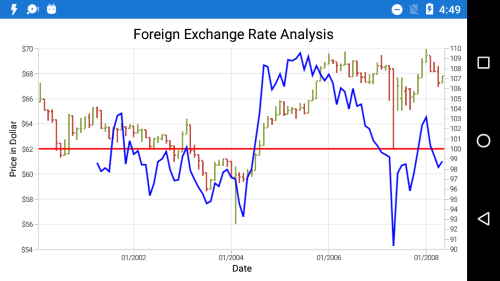## Stochastic indicator

This indicator is used to measure the range and momentum of price movements. It contains `KPeriod` and `DPeriod` property defining the ‘K’ percentage and ‘D’ percentage respectively. No signal line in this indicator.
The `UpperLineColor`, `LowerLineColor` and `PeriodLineColor` property are used to define the color for the Stochastic indicator lines.
You can define `StochasticIndicator` using the following code example.

``````<chart:SfChart>
...
<chart:SfChart.TechnicalIndicators>
<chart:StochasticIndicator ItemsSource="{Binding TechnicalIndicatorData}" Period="14" SignalLineColor="Yellow" UpperLineColor="Red" LowerLineColor="Teal" PeriodLineColor="DarkBlue" KPeriod="8" DPeriod="5" XBindingPath="XValue" High="High" Low="Low" Open="Open" Close="Close"/>
</chart:SfChart.TechnicalIndicators>
</chart:SfChart>``````
``````SfChart chart = new SfChart()
{
...
TechnicalIndicators =
{
new StochasticIndicator()
{
Period = 14,
SignalLineColor = Color.Yellow,
UpperLineColor = Color.Red,
LowerLineColor = Color.Teal,
PeriodLineColor = Color.DarkBlue,
KPeriod = 8,
DPeriod = 5,
ItemsSource = viewModel.TechnicalIndicatorData,
XBindingPath = "XValue",
Open = "Open",
High = "High",
Low = "Low",
Close = "Close",
}
}
};``````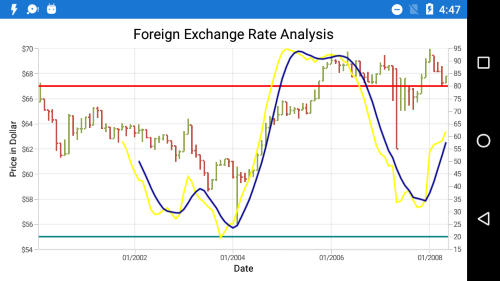## Exponential moving average indicator

The `ExponentialMovingAverageIndicator` is similar to `SimpleMovingAverageIndicator` and this can be defined using the following code examples.

``````<chart:SfChart>
...
<chart:SfChart.TechnicalIndicators>
<chart:ExponentialMovingAverageIndicator ItemsSource="{Binding TechnicalIndicatorData}" Period="14" SignalLineColor="Blue" XBindingPath="XValue" High="High" Low="Low" Open="Open" Close="Close"/>
</chart:SfChart.TechnicalIndicators>
</chart:SfChart>``````
``````SfChart chart = new SfChart()
{
...
TechnicalIndicators =
{
new ExponentialMovingAverageIndicator()
{
Period = 14,
SignalLineColor = Color.Blue,
ItemsSource = viewModel.TechnicalIndicatorData,
XBindingPath = "XValue",
Open = "Open",
High = "High",
Low = "Low",
Close = "Close",
}
}
};``````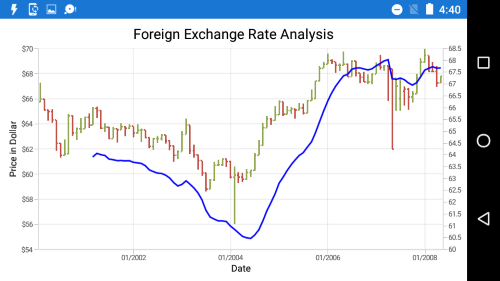## Triangular moving average indicator

A Triangular moving average is simply a double-smoothed simple moving average of data calculated over a period of time where the middle portion of the data has more weight.
The `TriangularMovingAverageIndicator` can be defined as in the following code example.

``````<chart:SfChart>
...
<chart:SfChart.TechnicalIndicators>
<chart:TriangularMovingAverageIndicator ItemsSource="{Binding TechnicalIndicatorData}" Period="14" SignalLineColor="Blue" XBindingPath="XValue" High="High" Low="Low" Open="Open" Close="Close"/>
</chart:SfChart.TechnicalIndicators>
</chart:SfChart>``````
``````SfChart chart = new SfChart()
{
...
TechnicalIndicators =
{
new TriangularMovingAverageIndicator()
{
Period = 14,
SignalLineColor = Color.Blue,
ItemsSource = viewModel.TechnicalIndicatorData,
XBindingPath = "XValue",
Open = "Open",
High = "High",
Low = "Low",
Close = "Close",
}
}
};``````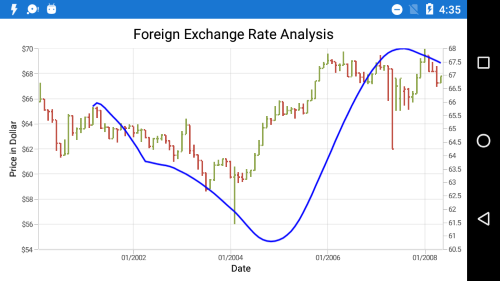## Bollinger band indicator

This indicator also having `UpperLineColor`, `LowerLineColor` and `SignalLineColor` property for defining the brushes for the indicator lines.

Also, we can specify standard deviation values for BollingerBand indicator using `StandardDeviation` property.
You can define the `BollingerBandIndicator` using the following code example.

``````<chart:SfChart>
...
<chart:SfChart.TechnicalIndicators>
<chart:BollingerBandIndicator ItemsSource="{Binding TechnicalIndicatorData}" Period="14" SignalLineColor="Blue" UpperLineColor="Red" LowerLineColor="Teal" XBindingPath="XValue" High="High" Low="Low" Open="Open" Close="Close"/>
</chart:SfChart.TechnicalIndicators>
</chart:SfChart>``````
``````SfChart chart = new SfChart()
{
...
TechnicalIndicators =
{
new BollingerBandIndicator()
{
Period = 14,
SignalLineColor = Color.Blue,
UpperLineColor = Color.Red,
LowerLineColor = Color.Teal,
ItemsSource = viewModel.TechnicalIndicatorData,
XBindingPath = "XValue",
Open = "Open",
High = "High",
Low = "Low",
Close = "Close",
}
}
};``````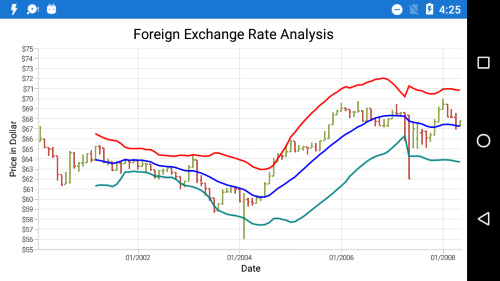## MACD indicator

This is mostly using indicator having `ShortPeriod` and `LongPeriod` for defining the motion of the indicator.
Also you can draw `Line`, `Histogram` MACD or `Both` using the `MACDType` property, which defines the type of MACD to be drawn.

The `MACDLineColor` property is used to define the color for the MACD line and the `HistogramLineColor` property is used to define the color for the MACD histogram.
You can specify the MACD indicator using the following code example.

``````<chart:SfChart>
...
<chart:SfChart.TechnicalIndicators>
<chart:MACDIndicator ItemsSource="{Binding TechnicalIndicatorData}" MACDType="Both" ShortPeriod="2" LongPeriod="10" Trigger="14" SignalLineColor="Blue" HistogramColor="LightSkyBlue" MACDLineColor="Orange" XBindingPath="XValue" High="High" Low="Low" Open="Open" Close="Close"/>
</chart:SfChart.TechnicalIndicators>
</chart:SfChart>``````
``````SfChart chart = new SfChart()
{
...
TechnicalIndicators =
{
new MACDIndicator()
{
MACDType = MACDType.Both,
ShortPeriod = 2,
LongPeriod = 10,
Trigger = 14,
SignalLineColor = Color.Blue,
HistogramColor = Color.LightSkyBlue,
MACDLineColor = Color.Orange,
ItemsSource = viewModel.TechnicalIndicatorData,
XBindingPath = "XValue",
Open = "Open",
High = "High",
Low = "Low",
Close = "Close",
}
}
};``````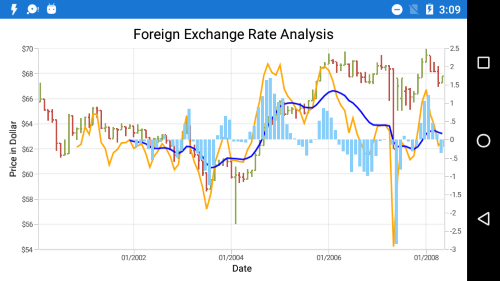NOTE

You can refer to our Xamarin Charts feature tour page for its groundbreaking feature representations. You can also explore our Xamarin.Forms Charts example to knows various chart types and how to easily configured with built-in support for creating stunning visual effects.